Numbers, representations of

The totality of ways of representing natural numbers. In any number system, certain symbols (words or signs) denote specific numbers, called nodal numbers, while the other numbers are (algorithmically) obtained by certain operations from the nodal numbers. Number systems vary in their choice of nodal numbers and in their methods of forming algorithmic numbers; as written notations of numerical symbols began to appear, so number systems began to vary in the character of their numerical signs and in the principles governing the form in which they were written.

For example, the ancient Babylonians used 1, 10 and 60 as nodal numbers; the Maoris (the initial inhabitants of New Zealand) used 1, 11,,. In the Roman number system the nodal numbers are 1, 5, 10, 50, 100, 500, 1000, represented respectively by the signs I, V, X, L, C, D, M (cf. Roman numerals).

Number systems in which the algorithmic numbers are formed by grouping nodal numbers together are called additive systems. Thus, in ancient Egyptian (hieroglyphic) notation, the numbers 1, 2, 3, 4, 5, 6, 7, 8, 9, 10, 19, 40 were represented, respectively, by the symbolsThe same numbers in Roman numerals are written I, II, III, IV, V, VI, VII, VIII, IX, X, XIX, XL. In this number system, the algorithmic numbers are obtained by the addition and subtraction of nodal numbers. The English method of expressing numerals is a clear example of the additive-multiplicative method of forming algorithmic numbers, for example: three hundred fifty seven.

In certain number systems, called alphabetical systems, numbers are represented by the same symbols as letters, plus other signs, e.g. dashes. Thus, the ancient Greeks denoted the numbers 1 to 9, as well as all the tens and hundreds, by sequences of letters of the alphabet, combined with dashes. For example, the numbers 803, 833 and 83 were written thus: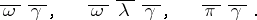Alphabetical representations of numbers were used by the Slavs and many other peoples (cf. Slavic numerals).

Number systems are called non-positional if every sign used in the notation of any number has only one value. If the value of each sign depends on its position within the notation, then the system is called positional. The Roman number system is non-positional. Any number in the Babylonian number system could be written as a combination of two signs: a vertical wedge and a wide-angle wedge (see the example below). These signs were formed into groups from one to nine in the case of the vertical wedges, and from one to five in the case of the wide-angle wedges. The vertical wedge could represent 10 and the product of 10 and any power of the number 60. The sequential order of the digit positions was the same as it is presently. Thus,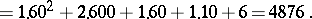Since the Babylonian system had no sign corresponding to no sequence (our zero), there was no guarantee that the notation of a number could be read in only one way. The exact meaning of the notation could normally be established from the context. This type of number system is therefore called a non-absolute positional system. The ancient Babylonians did subsequently introduce a special sign corresponding to our zero. The modern decimal system is positional.

All known positional number systems are additive-multiplicative systems. The positional principle of notation of numbers in these systems is explained by the following theorem of elementary number theory.

Letand let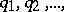be a sequence of natural numbers greater than one. Then for any natural numberthere is one and only one natural numberfor which the equation(1)

has a solution in integers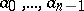, such that(2)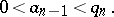Given this, only one ordered set (tuple)(3)

of integers with condition (2) satisfies condition (1).

In the Babylonian number system,,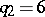,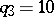,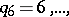etc. In the system of the Maya Indians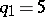,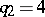,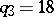,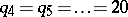.

A number system in which all terms of the sequenceare equal to one and the same numberand in which every number from 0 to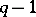is denoted by a specific symbol is called a-ic number system or a positional number system with basis. In a-ic system, every natural number is denoted by a sequence of the symbols shown. In order to add and multiply the numbers in a-ic system, it is sufficient to have addition and multiplication tables for all numbers from 0 to.